# P 6 Differential Equations Copyright Cengage Learning All

• Slides: 21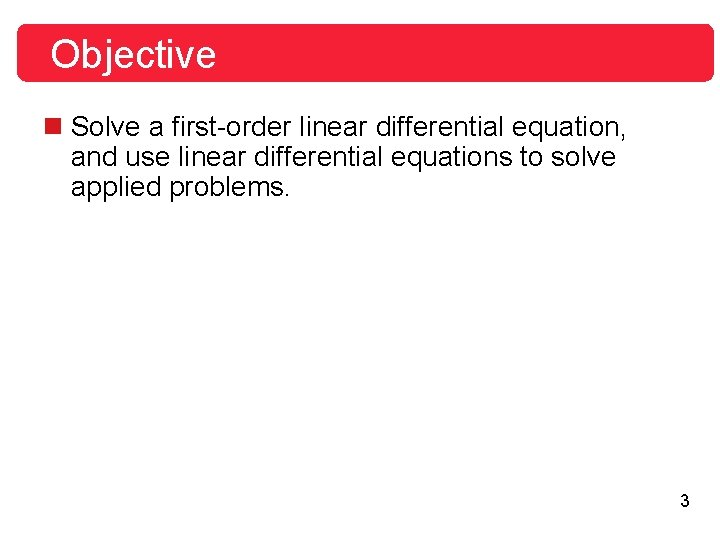Objective n Solve a first-order linear differential equation, and use linear differential equations to solve applied problems. 3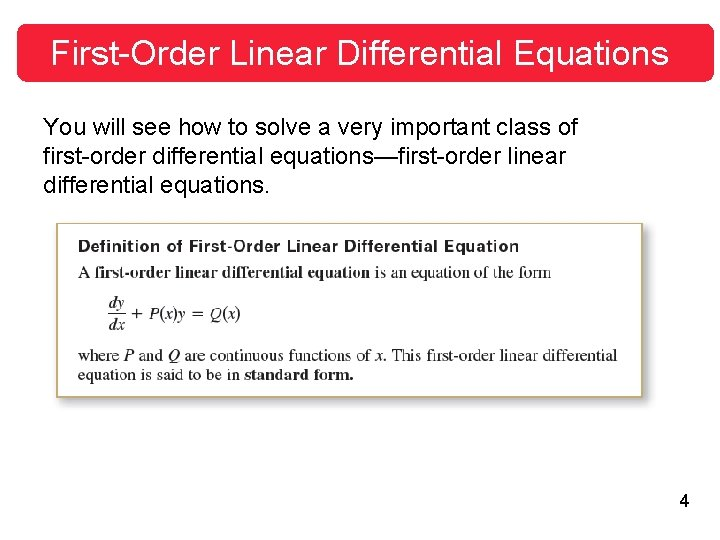First-Order Linear Differential Equations You will see how to solve a very important class of first-order differential equations—first-order linear differential equations. 4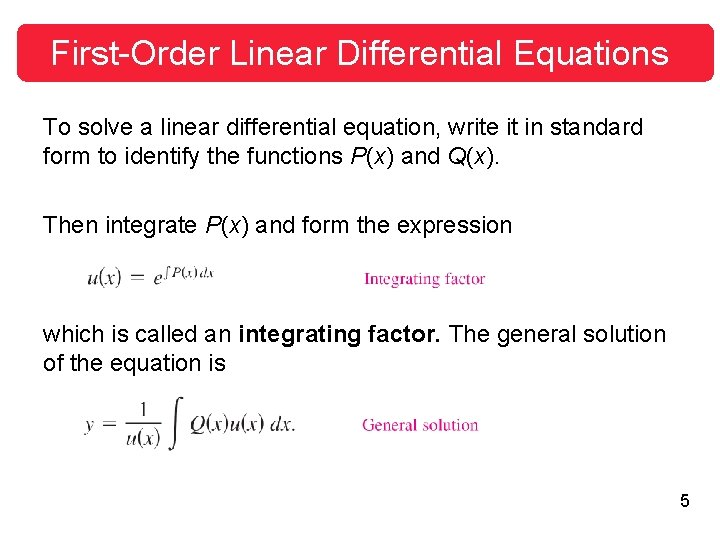First-Order Linear Differential Equations To solve a linear differential equation, write it in standard form to identify the functions P(x) and Q(x). Then integrate P(x) and form the expression which is called an integrating factor. The general solution of the equation is 5First-Order Linear Differential Equations It is instructive to see why the integrating factor helps solve a linear differential equation of the form y' = P(x)y = Q(x). When both sides of the equation are multiplied by the integrating factor the left side becomes the derivative of a product. Integrating both sides of this second equation and dividing by u(x) produce the general solution. 6Example 1 – Solving a Linear Differential Equation Find the general solution of y' + y = ex. Solution: For this equation, P(x) = 1 and Q(x) = ex. So, the integrating factor is 7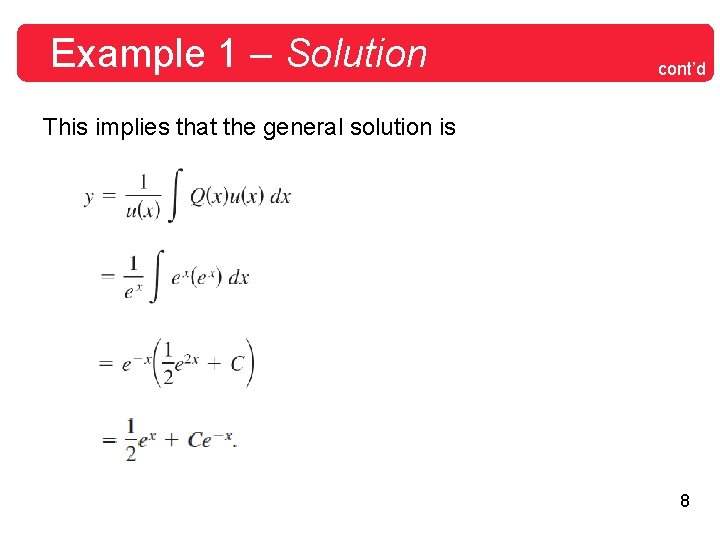Example 1 – Solution cont’d This implies that the general solution is 8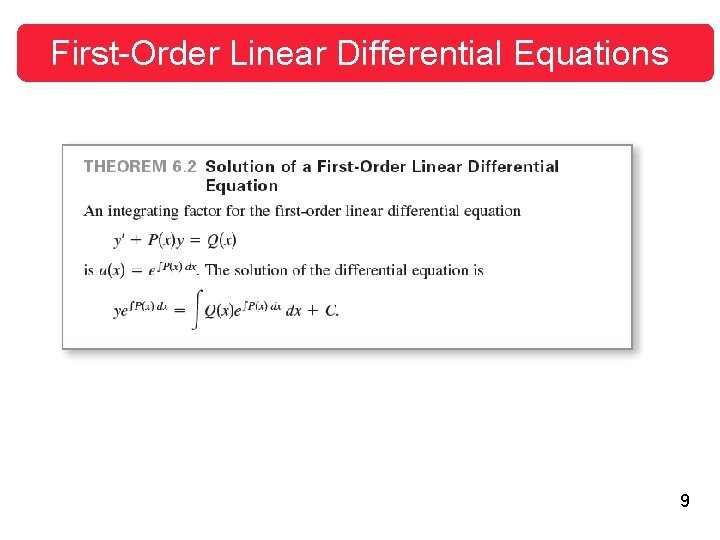First-Order Linear Differential Equations 9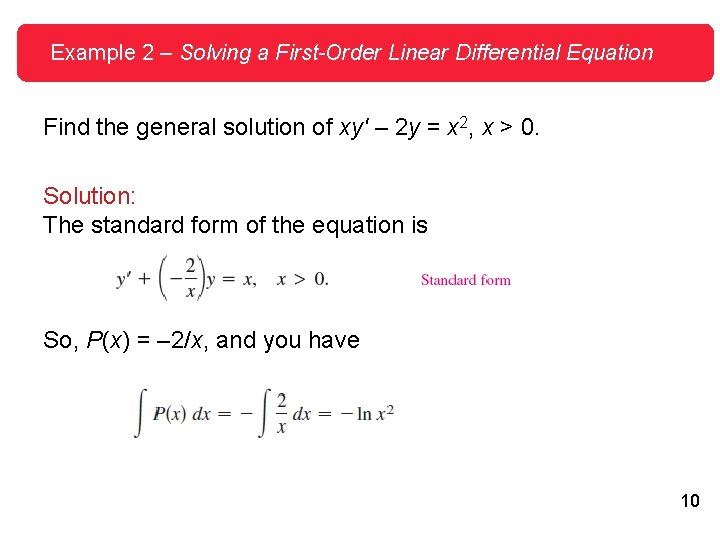Example 2 – Solving a First-Order Linear Differential Equation Find the general solution of xy' – 2 y = x 2, x > 0. Solution: The standard form of the equation is So, P(x) = – 2/x, and you have 10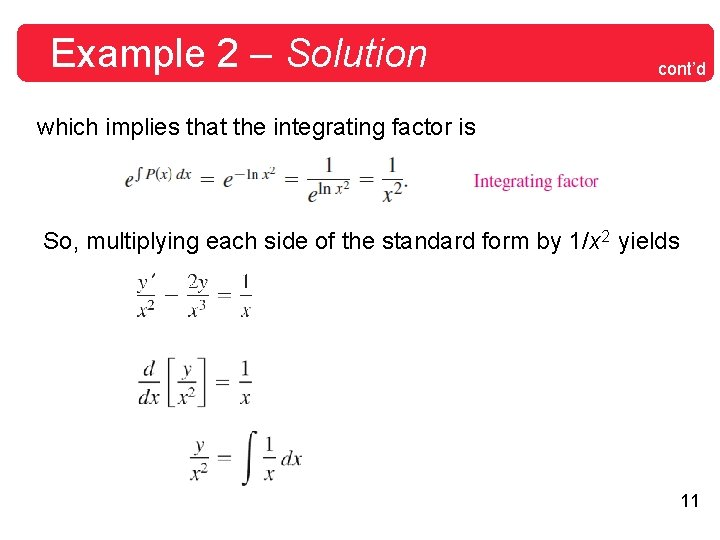Example 2 – Solution cont’d which implies that the integrating factor is So, multiplying each side of the standard form by 1/x 2 yields 11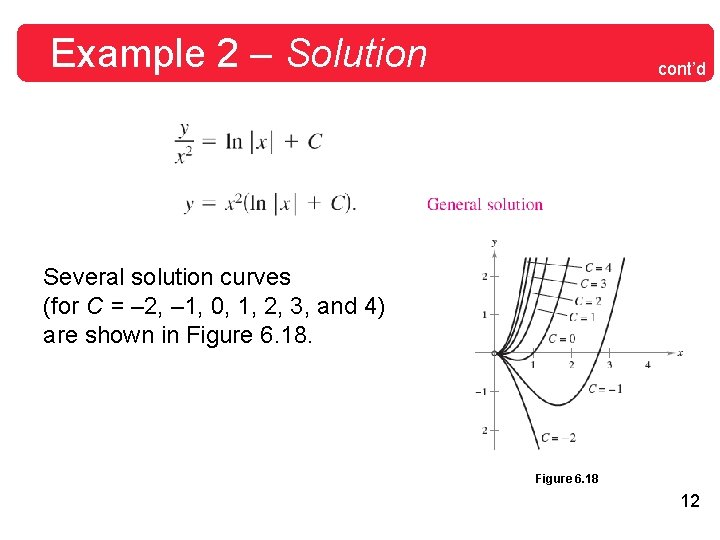Example 2 – Solution cont’d Several solution curves (for C = – 2, – 1, 0, 1, 2, 3, and 4) are shown in Figure 6. 18 12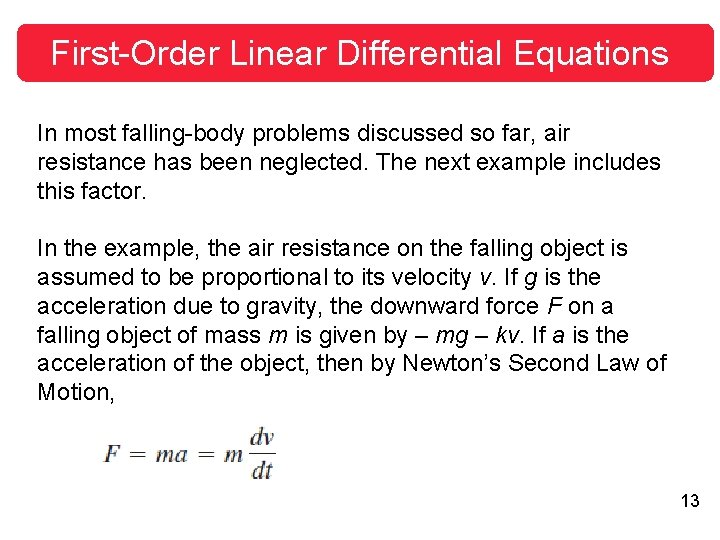First-Order Linear Differential Equations In most falling-body problems discussed so far, air resistance has been neglected. The next example includes this factor. In the example, the air resistance on the falling object is assumed to be proportional to its velocity v. If g is the acceleration due to gravity, the downward force F on a falling object of mass m is given by – mg – kv. If a is the acceleration of the object, then by Newton’s Second Law of Motion, 13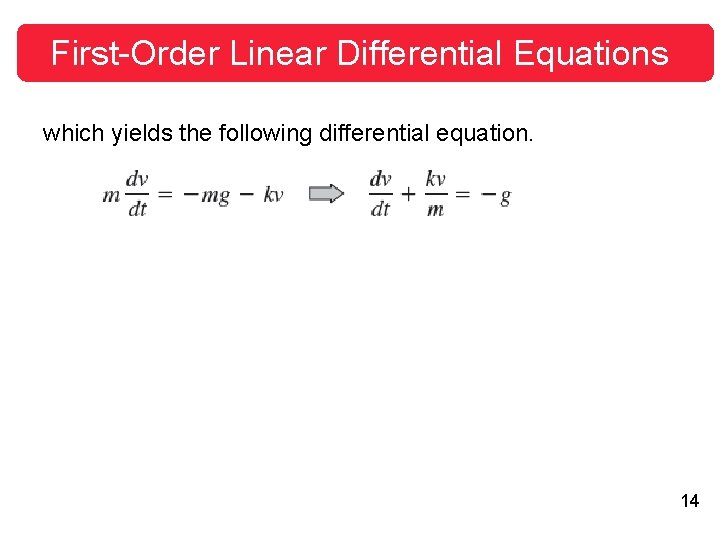First-Order Linear Differential Equations which yields the following differential equation. 14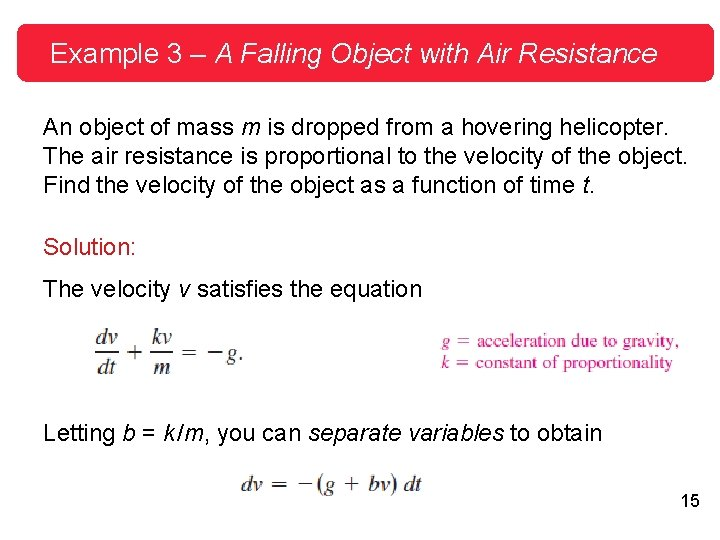Example 3 – A Falling Object with Air Resistance An object of mass m is dropped from a hovering helicopter. The air resistance is proportional to the velocity of the object. Find the velocity of the object as a function of time t. Solution: The velocity v satisfies the equation Letting b = k /m, you can separate variables to obtain 15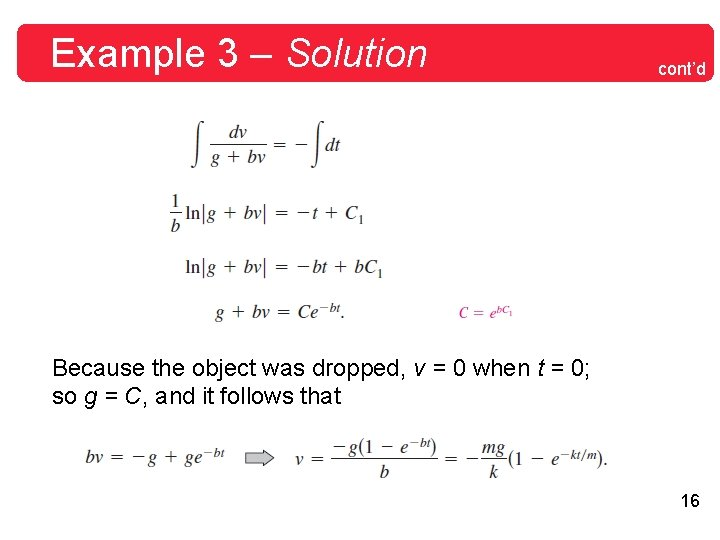Example 3 – Solution cont’d Because the object was dropped, v = 0 when t = 0; so g = C, and it follows that 16Example 5 – A Mixture Problem A tank contains 50 gallons of a solution composed of 90% water and 10% alcohol. A second solution containing 50% water and 50% alcohol is added to the tank at the rate of 4 gallons per minute. As the second solution is being added, the tank is being drained at a rate of 5 gallons per minute, as shown in Figure 6. 20. The solution in the tank is stirred constantly. How much alcohol is in the tank after 10 minutes? Figure 6. 20 17Example 5 – Solution Let y be the number of gallons of alcohol in the tank at any time t. You know that y = 5 when t = 0. Because the number of gallons of solution in the tank at any time is 50 – t, and the tank loses 5 gallons of solution per minute, it must lose gallons of alcohol per minute. Furthermore, because the tank is gaining 2 gallons of alcohol per minute, the rate of change of alcohol in the tank is 18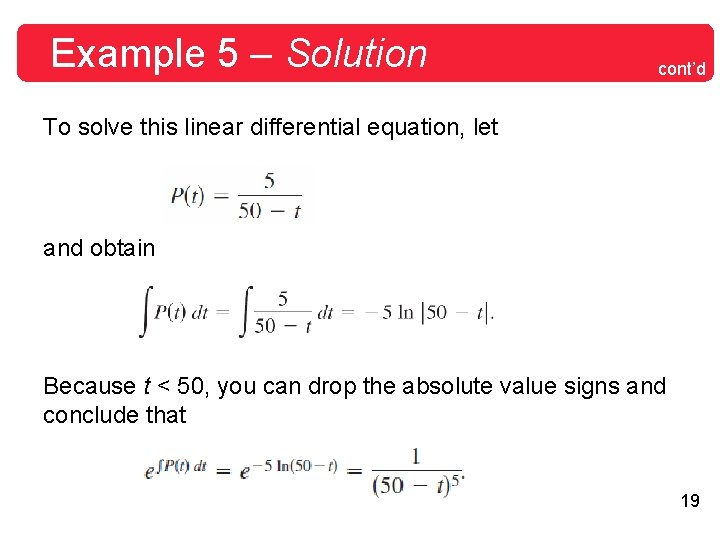Example 5 – Solution cont’d To solve this linear differential equation, let and obtain Because t < 50, you can drop the absolute value signs and conclude that 19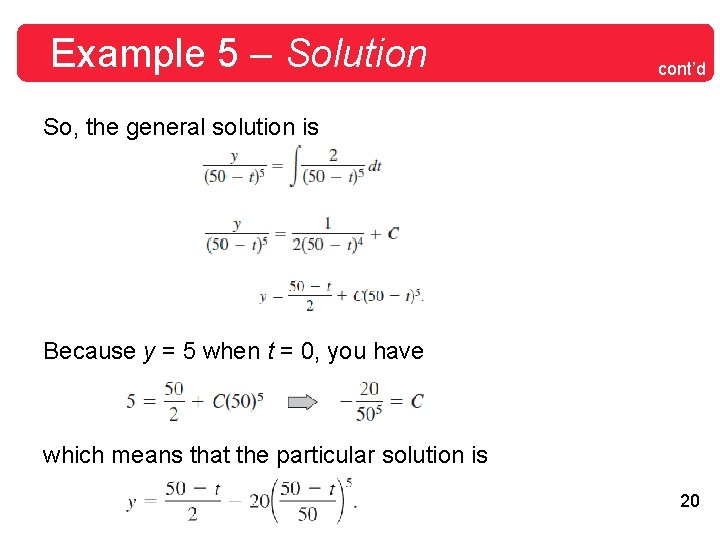Example 5 – Solution cont’d So, the general solution is Because y = 5 when t = 0, you have which means that the particular solution is 20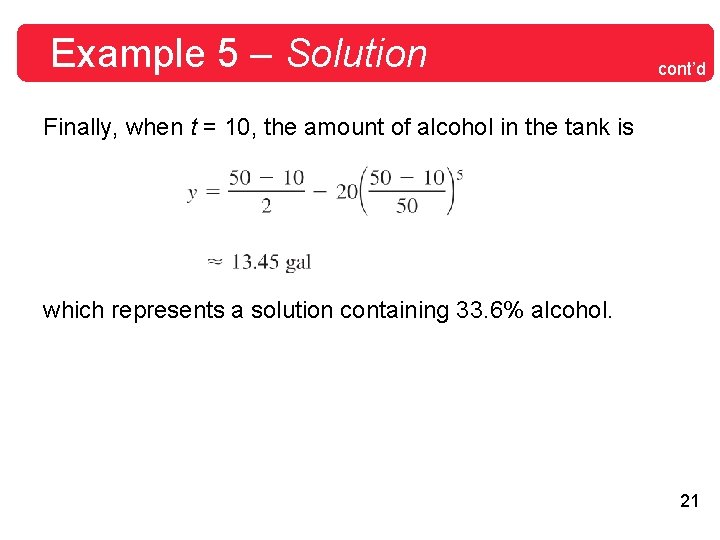Example 5 – Solution cont’d Finally, when t = 10, the amount of alcohol in the tank is which represents a solution containing 33. 6% alcohol. 21